The .Wat ness is open for testing! http://watness.pwni.ng:7744/

When it came out in 2016, the Witness impressed with its ability to gradually teach new players the rules of the game by simply having them play it. With the .Wat ness, we take this just a small step further.

## How to solve#

First of all, this write-up has a lot of guesses.

### Super easy guesses#

If you’re a ’the witness’ player, you can easily guess four symbols: (Read this with the guide)

• Multicolored squares in the Witness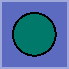• Suns in the Witness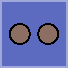• Tetris blocks in the Witness• Triangles in the WitnessWait, why the guide does not explain triangles? You need to draw a path exactly passes n edges of the block with n triangles. In The .Wat ness, it is n blue blocks.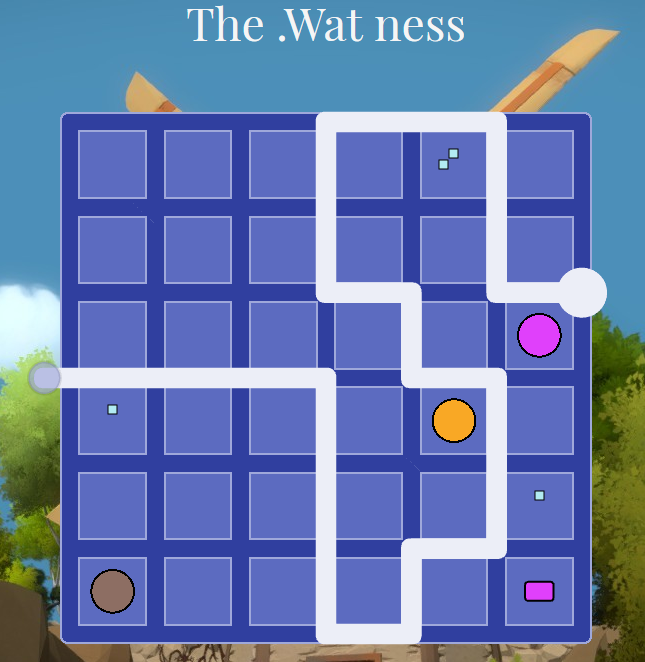But what are those?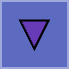We need to figure them out…

### Reversing#

By reading src/constraints.ts, you can know there are total 6 constraints and Constraint5 is reversed triangles, and Constraint6 is round-edged rectangle.

By reading problem/common/src/bootstrap.ts, you can see four parameters are passed to fn_assembly/index/checkAll. (In the code, it is this.module.checkAll.) If checkAll returns an empty array, it is the answer. So, what are those four parameters?

 - mtx (parameter 1) : array_BoardMatrixElement
- parts (parameter 2) : array_PartitionListElement
- horizontalPathSegments (parameter 3) : array_i32
- verticalPathSegments (parameter 4): array_i32


The first parameter describes the symbols on the board.

If the board is partitioned by the input path, partition information is passed by the second parameter.

The third and fourth parameter have which edge is passed by the input path. So, the third one will be kinda 7×6 matrix, and the fourth one will be 6×7 matrix.

I’ve run wabt wasm2c to read the wasm file.

checkAll calls Constraint{i}_check functions. Let’s see Constraint6_check.
When reading the code, p{i} is the ith parameter, l{i} is a local variable, and l{i} is a temporal variable. Just understand l{i} as non-volatile registers, and i{i} as volatile registers.

static u32 assembly_index_Constraint6_check(u32 p0, u32 p1, u32 p2, u32 p3) {
u32 l4 = 0, l5 = 0, l6 = 0, l7 = 0, l8 = 0;
FUNC_PROLOGUE;
u32 i0, i1, i2, i3;
i0 = 0u;
i1 = 0u;
i0 = _lib_array_Array_Constraint__constructor(i0, i1);
l4 = i0;
i0 = 0u;
l5 = i0;
L1:
i0 = l5;
i1 = p0;
l6 = i1;
i1 = l6;
i1 = i32_load((&memory), (u64)(i1 + 4));
i0 = (u32)((s32)i0 < (s32)i1);
i0 = !(i0);
if (i0) {goto B0;}
i0 = 0u;
l6 = i0;
L4:
i0 = l6;
i1 = p0;
i2 = l5;
i1 = _lib_array_Array_Array_BoardMatrixElement_____get(i1, i2);
l7 = i1;
i1 = l7;
i1 = i32_load((&memory), (u64)(i1 + 4));
i0 = (u32)((s32)i0 < (s32)i1);
i0 = !(i0);
if (i0) {goto B3;}
i0 = p0;
i1 = l5;
i0 = _lib_array_Array_Array_BoardMatrixElement_____get(i0, i1);
i1 = l6;
i0 = _lib_array_Array_BoardMatrixElement____get(i0, i1);
i0 = i32_load((&memory), (u64)(i0 + 4));
i1 = 0u;
i0 = i0 != i1;
l7 = i0;
if (i0) {
i0 = p0;
i1 = l5;
i0 = _lib_array_Array_Array_BoardMatrixElement_____get(i0, i1);
i1 = l6;
i0 = _lib_array_Array_BoardMatrixElement____get(i0, i1);
i0 = i32_load((&memory), (u64)(i0 + 4));
i0 = i32_load((&memory), (u64)(i0 + 12));
i1 = 2344u;
i0 = assembly_index_streq(i0, i1);
} else {
i0 = l7;
}
if (i0) {
i0 = p0;
i1 = l5;
i0 = _lib_array_Array_Array_BoardMatrixElement_____get(i0, i1);
i1 = l6;
i0 = _lib_array_Array_BoardMatrixElement____get(i0, i1);
l7 = i0;
i0 = p2;
i1 = l5;
i0 = _lib_array_Array_Array_i32_____get(i0, i1);
i1 = l6;
i0 = _lib_array_Array_i32____get(i0, i1);
i1 = p2;
i2 = l5;
i3 = 1u;
i2 += i3;
i1 = _lib_array_Array_Array_i32_____get(i1, i2);
i2 = l6;
i1 = _lib_array_Array_i32____get(i1, i2);
i0 += i1;
i1 = p3;
i2 = l5;
i1 = _lib_array_Array_Array_i32_____get(i1, i2);
i2 = l6;
i1 = _lib_array_Array_i32____get(i1, i2);
i0 += i1;
i1 = p3;
i2 = l5;
i1 = _lib_array_Array_Array_i32_____get(i1, i2);
i2 = l6;
i3 = 1u;
i2 += i3;
i1 = _lib_array_Array_i32____get(i1, i2);
i0 += i1;
l8 = i0;
i0 = l8;
i1 = 0u;
i0 = i0 != i1;
if (i0) {
i0 = l4;
i1 = p0;
i2 = l5;
i1 = _lib_array_Array_Array_BoardMatrixElement_____get(i1, i2);
i2 = l6;
i1 = _lib_array_Array_BoardMatrixElement____get(i1, i2);
i1 = i32_load((&memory), (u64)(i1 + 4));
i0 = _lib_array_Array_Constraint__push(i0, i1);
}
}
i0 = l6;
i1 = 1u;
i0 += i1;
l6 = i0;
goto L4;
UNREACHABLE;
B3:;
i0 = l5;
i1 = 1u;
i0 += i1;
l5 = i0;
goto L1;
UNREACHABLE;
B0:;
i0 = l4;
FUNC_EPILOGUE;
return i0;
}


L1 and L2 are loop labels. Each uses l5 and l6 as an iterator.
The key part is Line 55-104. It checks whether any edge around the block is passed by the input path. If yes, it will return those blocks as array_Constraint.

The return value should be an empty array, so round-edged rectangle symbols are like blue blocks symbols with zero blue blocks. We need to avoid four edges around round-edged rectangles, regardless of its color!

Then, what are reversed triangles? Well, I don’t know.

It’s still solvable without knowing it. Just try all the possible paths around reversed triangles. One of them will be a solution.

### Solving#

Just do by your hands. If it seems hard to solve, just press F5. I solved all the stages within 2 minutes.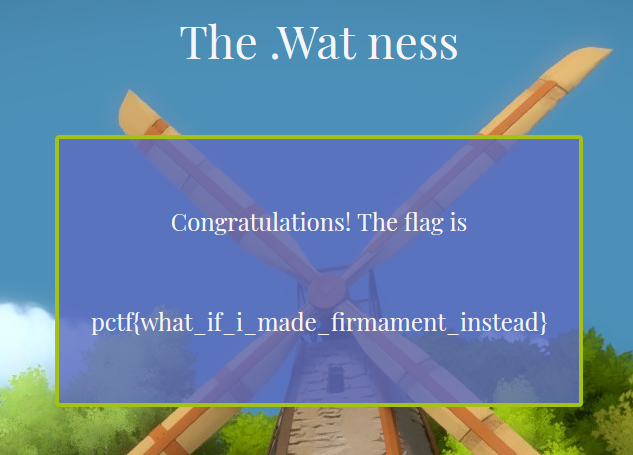The flag is pctf{what_if_i_made_firmament_instead}.

BTW, I solved all the puzzles in the Witness.